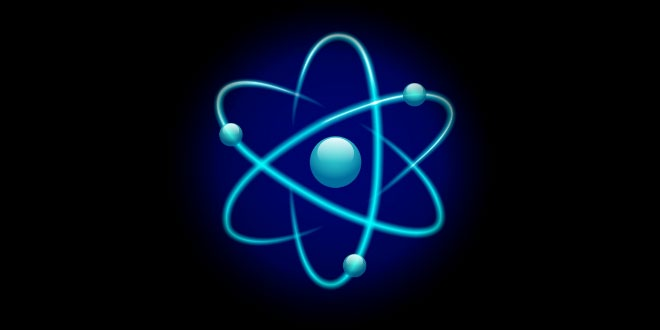Thursday , September 29 2022# 9th Class (CBSE) Science: Structure of the Atom

#### Question: What is an election? State its relative mass and charge.

Answer: The negatively charged particle found in the atoms of all elements is known as electron. The relative mass of an election is 1/1840 u & charge is – 1 (minus one).

#### Question: What is the absolute mass & charge of an election?

Absolute Mass      →  9 × 1028 g

Absolute Charge  →  1.6 × 1019 coulomb

#### Question: What is the absolute mass & charge of a proton?

Absolute Mass      →  1.6 × 1024 g

Absolute Charge  →  1.6 × 1019 coulomb

#### Question: What are canal rays?

Answer: Canal rays are positively charged radiations which led to the discovery of positively charged sub-atomic particle called proton.

#### Question: If an atom contains one electron and one proton, will it carry any charge or not?

Answer: The atom will be electrically neutral as one –ve charge balances one +ve charge.

#### Question: On the basis of Thomson’s model of an atom, explain how the atom is neutral as a whole.

Answer: According to Thomson’s model of an atom

1. An atom consists of a positively charged sphere and the electrons are embedded in it
2. The negative and positive charges are equal in magnitude. So the atom is electrically neutral

#### Question: On the basis of Rutherford’s model of an atom, which sub-atomic particle is present in the nucleus of an atom?

Answer: As per Rutherford’s model of an atom, the protons which are positively charged are present in the nucleus of an atom.

#### Question: What do you think would be the observation if the a-particle scattering experiment is carried out using a foil of a metal other than gold?

Answer: On using any metal foil, the observations of the a-particle scattering experiment would remain the same as all atoms would have same structure.

#### Question: Name the three sub-atomic particles of an atom.

Answer: The sub-atomic particles of an atom are:

Protons → Positively charged
Electrons → Negatively charged
Neutrons → No charge

#### Question: Helium atom has an atomic mass of 4 u and two protons in its nucleus. How many neutrons does it have?

Atomic mass of He = 4 u.

Atomic mass = No. of protons + No. of neutrons

∴                     4 = 2 +  no. of neutrons

∴  No. of neutrons = 4 – 2 = 2

Helium atom has 2 neutrons.

#### Question: Write the distribution of electrons in carbon and sodium atoms.

Write the distribution of electrons in carbon and sodium atoms.

Carbon                Atomic Number = 6

∴                                 No. of Protons = 6

and Number of protons = Number of electrons

∴            Distribution of electrons = KL

24

Sodium               Atomic Number = 11

∴                                 No. of Protons = 11 = No. of electrons

and Number of protons = Number of electrons

∴            Distribution of electrons = K L M

2 8 1

#### Question: If K and L shells of an atom are full, then what would be the total number of electrons in the atom?

Answer: K shell can hold 2 electrons and L shell can hold 8 electrons.When both the shells are full, there will be (8 + 2) 10 electrons in the atom.

#### Question: How will you find the valency of chlorine, sulphur and magnesium?

Answer: Valency is the combing capacity of an atom of an element.

Chlorine,                             Atomic Number = 17

∴                                                              Protons = 17, Electrons = 17

∴                                 Distribution of elections = K L M

2 8 7

Chlorine needs 1 electron to complete its outermost orbit/shell.

∴  Its valency is -1 (gains 1 electron).

Sulphur,                              Atomic number = 16

∴                                                             Protons = 16, Electrons = 16

∴                                Distribution of elections = K L M

2 8 6

Sulphur needs 2 electrons to complete its outermost shell.

∴      Its valency is -2 (gains 2 electron)

Magnesium,         Atomic number = 12

∴                                              Protons = 12, Electrons = 12

∴                                  Distribution of elections = K L M

2 8 2

Magnesium needs to donate 2 electrons from its outermost shell.

∴      Its valency needs to donate + 2 (gains 2 electron)

## Sources of Energy: 10th Science Chapter 14

Class: 10th Class Subject: Science Chapter: Chapter 14: Sources of Energy Quiz: – Questions MCQs: …